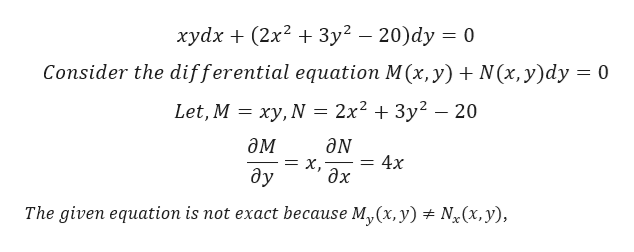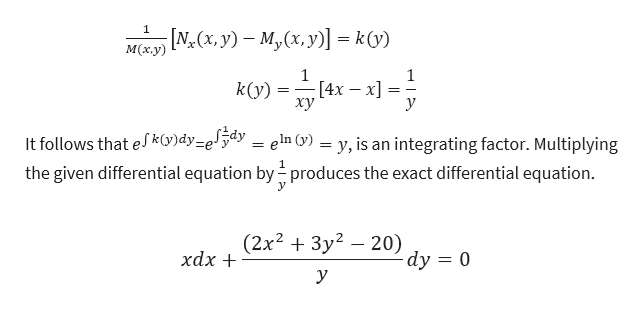# Solve the given initial value problem by using the method of exactness. xy dx+(2x2+3y2-20)dy=0, y(0)=1

Question
36 views

Solve the given initial value problem by using the method of exactness.

xy dx+(2x2+3y2-20)dy=0, y(0)=1

check_circle

Step 1

First identify M and N and check that the differential equation is exact.help_outlineImage Transcriptioncloseхуdx + (2x? + Зу? — 20)dy 0 Consider the differential equation M (x, y) N(x, y)dy 0 Let, M %3D ху, N 3D 2x2 + 3у2 — 20 дм ӘN х, дх 4x ду The given equation is not exact because My(x, y) Nr(x, y) fullscreen
Step 2

Hence, the given equation is not exact, then we can find the integrating factor and multiply the integrating factor and make it exact differential equation.help_outlineImage Transcriptionclose[N,(х, y) — м, (х, у)] -к0) M(х.у) 1 [4х — х] xy 1 k(у) у It follows that eK(V)dy=e'5dy = eln (y) = y, is an integrating factor. Multiplying the given differential equation by produces the exact differential equation. (2x2 + Зу? — 20) dy 0 хаx + y fullscreen
Step 3

By multiplying the integrating factor, the solution become exact,...

### Want to see the full answer?

See Solution

#### Want to see this answer and more?

Solutions are written by subject experts who are available 24/7. Questions are typically answered within 1 hour.*

See Solution
*Response times may vary by subject and question.
Tagged in

### Math